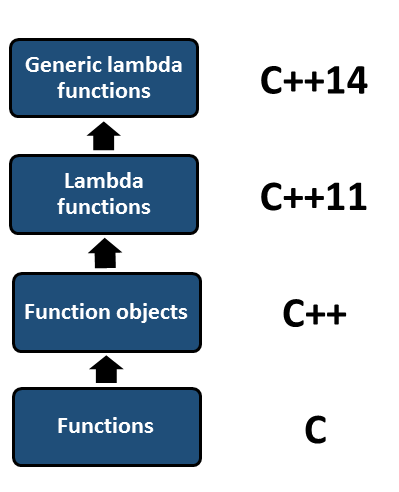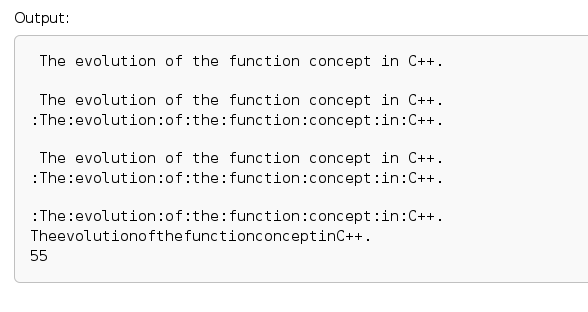# First-Class Functions

One of the characteristics of functional programming is first-class functions. First-class functions behave like data and are heavily used in the Standard Template Library.

## First-class functionsDo first-class functions behave like data? What does that mean?

First-class functions can be

• an argument of a function.

std::accumulate(vec.begin(), vec.end(), 1, []{ int a, int b}{ return a * b; })

• returned from a function.
``````       std::function<int(int, int)> makeAdd(){
return [](int a, int b){ return a + b; };
}
• stored in a variable.

One short remark on the second point. The function makeAdd returns the lambda function [](int a, int b){ return a + b; }. This function needs two int arguments and returns an int value: std::function<int(int,int)>. You can bind the return value of makeAdd to the generic function wrapper myAdd and execute it.

##Modernes C++ Mentoring

Be part of my mentoring programs:

• "Fundamentals for C++ Professionals" (open)
• "Design Patterns and Architectural Patterns with C++" (open)
• "C++20: Get the Details" (reopens December 2023)
• Do you want to stay informed about my mentoring programs: Subscribe via E-Mail.

My post Functional in C++11 and C++14: Dispatch Table and Generic Lambdas show which power lies in first-class functions. Have a look at the dispatch table in C++.

Function pointers are the first-class functions of the poor man. Even C has function pointers. C++ goes a few steps further. In particular, you can connect the evolution of the function concept with the evolution of C++.

## The evolution of the function concept

Simplified, evolution consists of four steps.C knows functions. C++ added function objects. These are objects which behave like functions because their call operator is overloaded. C++11 added lambda functions; C++14 made them generic. Each step made functions in C++ even more powerful.

• Functions => Function objects: Function objects can have a state contrary to functions. Therefore, they have a memory, and you can configure them.
• Function objects => Lambda functions: Lambda functions are usually implemented just in place of their usage. That improves the readability of the code, reduces your typing to its bare minimum, and gives the optimizer maximum insight into your code. Therefore, the optimizer has more opportunities to do its job.
• Lambda functions => Generic lambda functions: Generic lambda functions are similar to function templates but are much easier to implement. They can be widely used because, as closures, they capture their environment, and you can parametrize their type.

Admittedly, that was a lot of theory. Therefore, the example is following right now. Here are the four steps of the function concepts in C++.

 ``` 1 2 3 4 5 6 7 8 9 10 11 12 13 14 15 16 17 18 19 20 21 22 23 24 25 26 27 28 29 30 31 32 33 34 35 36 37 38 39 40 41 42 43 44 45 46 47 48 49 50 51 52 53 54 55 56 57 58 59 60 61 62 63 64 65 66 67 68 69 70 71 72 73 74 75``` ```// evolutionOfFunctions.cpp #include #include #include #include std::string addMe(std::string fir, std::string sec){ return fir + " " + sec; }; struct AddMe{ AddMe()= default; AddMe(std::string gl): glue(gl) {} std::string operator()(std::string fir, std::string sec) const { return fir + glue + sec; } std::string glue= " "; }; int main(){ std::vector myStrings={"The", "evolution", "of", "the", "function", "concept", "in", "C++."}; std::string fromFunc= std::accumulate(myStrings.begin(), myStrings.end(), std::string{}, addMe); std::cout << fromFunc << std::endl; std::cout << std::endl; std::string fromFuncObj= std::accumulate(myStrings.begin(), myStrings.end(), std::string{}, AddMe()); std::cout << fromFuncObj << std::endl; std::string fromFuncObj2= std::accumulate(myStrings.begin(), myStrings.end(), std::string{}, AddMe(":")); std::cout << fromFuncObj2 << std::endl; std::cout << std::endl; std::string fromLambdaFunc= std::accumulate(myStrings.begin(), myStrings.end(), std::string{}, [](std::string fir, std::string sec){ return fir + " " + sec; }); std::cout << fromLambdaFunc << std::endl; std::string glue=":"; auto lambdaFunc= [glue](std::string fir, std::string sec){ return fir + glue + sec; }; std::string fromLambdaFunc2= std::accumulate(myStrings.begin(), myStrings.end(), std::string{}, lambdaFunc); std::cout << fromLambdaFunc2 << std::endl; std::cout << std::endl; std::string fromLambdaFuncGeneric= std::accumulate(myStrings.begin(), myStrings.end(), std::string{}, [glue](auto fir, auto sec){ return fir + glue + sec; }); std::cout << fromLambdaFuncGeneric << std::endl; auto lambdaFuncGeneric= [](auto fir, auto sec){ return fir + sec; }; auto fromLambdaFuncGeneric1= std::accumulate(myStrings.begin(), myStrings.end(), std::string{}, lambdaFuncGeneric); std::cout << fromLambdaFuncGeneric1 << std::endl; std::vector myInts={1, 2, 3, 4, 5, 6, 7, 8, 9, 10}; auto fromLambdaFuncGeneric2= std::accumulate(myInts.begin(), myInts.end(), int{}, lambdaFuncGeneric); std::cout << fromLambdaFuncGeneric2 << std::endl; }```

The program begins with the function addMe (line 8 – 10), the function object AddMe (line 12 – 23), the lambda function (line 45 and 51), and the generic lambda function in line 59, 63, and 64. The program’s purpose is to concatenate the strings of the vector myString (line 27) in different ways with the help of the algorithm std::accumulate. Therefore, it is pretty comfortable that std::accumulate is a higher-order function (wait for my next post) and can accept first-class functions. I use a function pointer, a function object, and a (generic) lambda function as the first-class function.

I apply in line 29 the function pointer addMe. The function objects AddMe and AddMe(:) (lines 35 and 39) are more comfortable to use because I can parametrize the glue between the strings. The lambda functions offer the same comfort (lines 45 and 51). In particular, the lambda function lambdaFunc uses a copy of the variable glue (line 49). Therefore, the lambda function is a closure. The generic lambda function [glue](auto fir, auto sec){ return fir + glue + sec; }) (line 59) is the most interesting one. As closure, they also use variable glue. I apply the generic lambda function lambdaFuncGeneric to a std::vector<std::string> (line 27) and a std::vector<int> (line 69). In the case of std::vector<int>, the result is 55.

In the end, the output of the program.The evolution will not end with C++14. In C++20, we get high-probability coroutines, which generalize functions. Coroutines can be suspended and resumed. I will write a post about it. So, stay tuned.

### But that can be done more easily

For didactical reasons, I used std::accumulate to add pairs of elements together. That can be done much more straightforward – even for generic lambda functions – because std::accumulate has a simple version that needs no callable. The simple version adds their elements together, starting with the first element. Therefore, I can write line 65 a lot simpler: auto fromLambdaFuncGeneric1= std::accumulate(myStrings.begin(), myStrings.end(), std::string{}). The same holds for fromLambdaFuncGeneric2.

## What’s next?

I already mentioned it in the post. The classical counterpart to first-class functions is higher-order functions because they get first-class functions. The next post will be about higher-order functions—the following property of functional programming.

Thanks a lot to my Patreon Supporters: Matt Braun, Roman Postanciuc, Tobias Zindl, G Prvulovic, Reinhold Dröge, Abernitzke, Frank Grimm, Sakib, Broeserl, António Pina, Sergey Agafyin, Андрей Бурмистров, Jake, GS, Lawton Shoemake, Jozo Leko, John Breland, Venkat Nandam, Jose Francisco, Douglas Tinkham, Kuchlong Kuchlong, Robert Blanch, Truels Wissneth, Kris Kafka, Mario Luoni, Friedrich Huber, lennonli, Pramod Tikare Muralidhara, Peter Ware, Daniel Hufschläger, Alessandro Pezzato, Bob Perry, Satish Vangipuram, Andi Ireland, Richard Ohnemus, Michael Dunsky, Leo Goodstadt, John Wiederhirn, Yacob Cohen-Arazi, Florian Tischler, Robin Furness, Michael Young, Holger Detering, Bernd Mühlhaus, Matthieu Bolt, Stephen Kelley, Kyle Dean, Tusar Palauri, Dmitry Farberov, Juan Dent, George Liao, Daniel Ceperley, Jon T Hess, Stephen Totten, Wolfgang Fütterer, Matthias Grün, Phillip Diekmann, Ben Atakora, Ann Shatoff, Rob North, Bhavith C Achar, and Marco Parri Empoli.

Thanks, in particular, to Jon Hess, Lakshman, Christian Wittenhorst, Sherhy Pyton, Dendi Suhubdy, Sudhakar Belagurusamy, Richard Sargeant, Rusty Fleming, John Nebel, Mipko, Alicja Kaminska, Slavko Radman, and David Poole.

 My special thanks to EmbarcaderoMy special thanks to PVS-StudioMy special thanks to Tipi.buildMy special thanks to Take Up Code## Seminars

I’m happy to give online seminars or face-to-face seminars worldwide. Please call me if you have any questions.

### Standard Seminars (English/German)

Here is a compilation of my standard seminars. These seminars are only meant to give you a first orientation.

• C++ – The Core Language
• C++ – The Standard Library
• C++ – Compact
• C++11 and C++14
• Concurrency with Modern C++
• Design Pattern and Architectural Pattern with C++
• Embedded Programming with Modern C++
• Generic Programming (Templates) with C++

#### New

• Clean Code with Modern C++
• C++20

### Contact Me

Modernes C++ Mentoring,0 replies